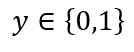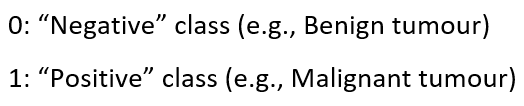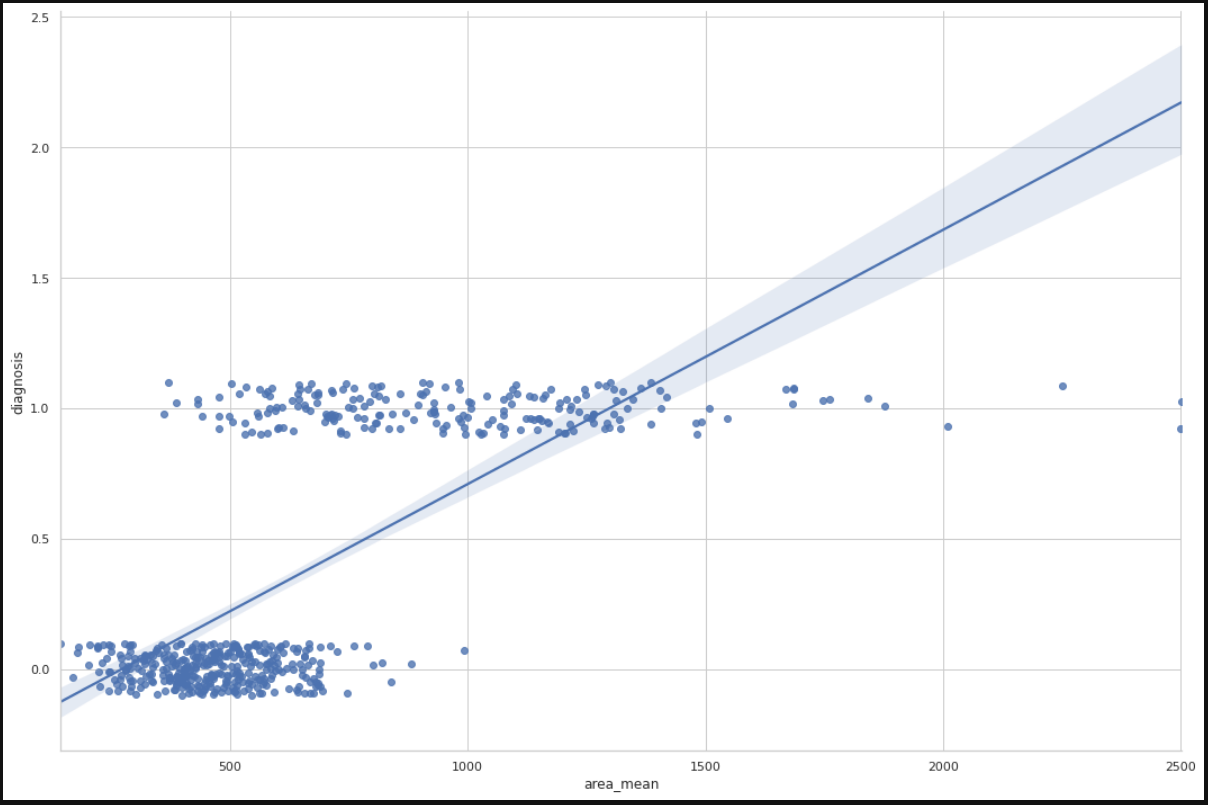1597051014

# Logistic Regression for Image Classification

You can probably figure out the image above — pretty easy, right! What if this task was assigned to a machine or your computer,will it still be an easy task.It’s not that simple for computers if you are wondering, as they do not the gift of vision and inbuilt human-like classification prowess. So it becomes one of the preliminary task to communicate with your machine which is obviously in the form of 1’s and 0’s , and if anyone has prior knowledge that an image can be represented in a form of binary digits which makes the communicating easier. With the recent advances in GPU capacity and convolutional neural network, computer vision has gained great acclaim. Computers now give us better accuracy than humans for images that are quite complex and have features that are not easily differentiable by naked eye, but then again computers have the prowess to figure out the details stringently. Let’s take a dive into the endless scope of machine learning by just exploring a sub-domain of its many application — classifying images.

### Introduction:

For the keeping things simple, we are going to use Logistic Regression for image classification. The data set for our study is one of the most popular handwritten digits know as MNIST dataset. In other words, given an image of a handwritten digit, we want to classify it as a 0, 1, 2, 3, … . Before we get started let’s import the necessary libraries.

#data-analysis #machine-learning #data-science

## Buddha Community1622617140

## Logistic Regression -Beginners Guide in Python - Analytics India Magazine

Most of the supervised learning problems in machine learning are classification problems. Classification is the task of assigning a data point with a suitable class. Suppose a pet classification problem. If we input certain features, the machine learning model will tell us whether the given features belong to a cat or a dog. Cat and dog are the two classes here. One may be numerically represented by 0 and the other by 1. This is specifically called a binary classification problem. If there are more than two classes, the problem is termed a multi-class classification problem. This machine learning task comes under supervised learning because both the features and corresponding class are provided as input to the model during training. During testing or production, the model predicts the class given the features of a data point.

This article discusses Logistic Regression and the math behind it with a practical example and Python codes. Logistic regression is one of the fundamental algorithms meant for classification. Logistic regression is meant exclusively for binary classification problems. Nevertheless, multi-class classification can also be performed with this algorithm with some modifications.

#developers corner #binary classification #classification #logistic regression #logit #python #regression #scikit learn #sklearn #statsmodels #tutorial1591903440

## Preprocessing your images for machine learning (image recognition)

During my studies at JKU there was a task for preprocessing images for a machine learning project. It is necessary to clean the raw images before using them in a learning algorithm, so thats why we create a pre-processing function. I think it can be quite useful for others as well so I want to share a bit of my approach. The file is structured in a way that it is easy to understand and also should have a tutorial-like effect.

#image-recognition #image #image-classification #machine-learning #image-processing1597019820

## Introduction:

In this article, I will be explaining how to use the concept of regression, in specific logistic regression to the problems involving classification. Classification problems are everywhere around us, the classic ones would include mail classification, weather classification, etc. All these data, if needed can be used to train a Logistic regression model to predict the class of any future example.

## Context:

1. Introduction to classification problems.
2. Logistic regression and all its properties such as hypothesis, decision boundary, cost, cost function, gradient descent, and its necessary analysis.
3. Developing a logistic regression model from scratch using python, pandas, matplotlib, and seaborn and training it on the Breast cancer dataset.
4. Training an in-built Logistic regression model from sklearn using the Breast cancer dataset to verify the previous model.

## Introduction to classification problems:

Classification problems can be explained based on the Breast Cancer dataset where there are two types of tumors (Benign and Malignant). It can be represented as:whereThis is a classification problem with 2 classes, 0 & 1. Generally, the classification problems have multiple classes say, 0,1,2 and 3.

## Dataset:

### Breast Cancer Wisconsin (Diagnostic) Data Set

Predict whether the cancer is benign or malignant

www.kaggle.com

1. Let’s import the dataset to a pandas dataframe:
``````import pandas as pd
``````

2. The following dataframe is obtained:

``````df.head()

![Image for post](https://miro.medium.com/max/1750/1*PPyiGgocvjHbgIcs9yTWTA.png)

``````

df.info()

### Data analysis:

Let us plot the mean area of the clump and its classification and see if we can find a relation between them.

``````import matplotlib.pyplot as plt
import seaborn as sns
from sklearn import preprocessing
label_encoder = preprocessing.LabelEncoder()
df.diagnosis = label_encoder.fit_transform(df.diagnosis)
sns.set(style = 'whitegrid')
sns.lmplot(x = 'area_mean', y = 'diagnosis', data = df, height = 10, aspect = 1.5, y_jitter = 0.1)
``````We can infer from the plot that most of the tumors having an area less than 500 are benign(represented by zero) and those having area more than 1000 are malignant(represented by 1). The tumors having a mean area between 500 to 1000 are both benign and malignant, therefore show that the classification depends on more factors other than mean area. A linear regression line is also plotted for further analysis.

#machine-learning #logistic-regression #regression #data-sceince #classification #deep learning1598352300

## Regression: Linear Regression

Machine learning algorithms are not your regular algorithms that we may be used to because they are often described by a combination of some complex statistics and mathematics. Since it is very important to understand the background of any algorithm you want to implement, this could pose a challenge to people with a non-mathematical background as the maths can sap your motivation by slowing you down.In this article, we would be discussing linear and logistic regression and some regression techniques assuming we all have heard or even learnt about the Linear model in Mathematics class at high school. Hopefully, at the end of the article, the concept would be clearer.

**Regression Analysis **is a statistical process for estimating the relationships between the dependent variables (say Y) and one or more independent variables or predictors (X). It explains the changes in the dependent variables with respect to changes in select predictors. Some major uses for regression analysis are in determining the strength of predictors, forecasting an effect, and trend forecasting. It finds the significant relationship between variables and the impact of predictors on dependent variables. In regression, we fit a curve/line (regression/best fit line) to the data points, such that the differences between the distances of data points from the curve/line are minimized.

#regression #machine-learning #beginner #logistic-regression #linear-regression #deep learning

1597565398

## Laravel 7/6 Image Validation

In this image validation in laravel 7/6, i will share with you how validate image and image file mime type like like jpeg, png, bmp, gif, svg, or webp before uploading image into database and server folder in laravel app.

https://www.tutsmake.com/image-validation-in-laravel/

#laravel image validation #image validation in laravel 7 #laravel image size validation #laravel image upload #laravel image validation max #laravel 6 image validation